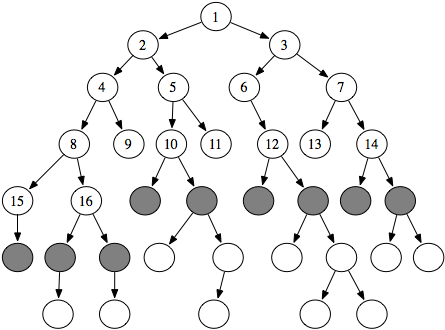In breadth-first search the frontier is implemented as a FIFO (first-in, first-out) queue. Thus, the path that is selected from the frontier is the one that was added earliest.

This approach implies that the paths from the start node are generated in order of the number of arcs in the path. One of the paths with the fewest arcs is selected at each stage.Figure 3.7: The order in which nodes are expanded in breadth-first search

Example 3.9: Consider the tree-shaped graph in Figure 3.7. Suppose the start node is the node at the top. In breadth-first search, as in depth-first search, the order in which the nodes are expanded does not depend on the location of the goal. The first sixteen nodes expanded are numbered in order of expansion in the figure. The shaded nodes are the nodes at the ends of the paths of the frontier after the first sixteen steps.
Example 3.10: Consider breadth-first search from o103 in the graph given in Figure 3.2. The only goal node is r123. Initially, the frontier is [⟨o103⟩]. This is extended by o103's neighbors, making the frontier [⟨o103,ts⟩, ⟨o103,b3⟩, ⟨o103,o109⟩]. These are the nodes one arc away from o013. The next three paths chosen are ⟨o103,ts⟩, ⟨o103,b3⟩, and ⟨o103,o109⟩, at which stage the frontier contains
[⟨o103,ts,mail⟩,⟨o103,b3,b1⟩,⟨o103,b3,b4⟩, ⟨o103,o109,o111⟩, ⟨o103,o109,o119⟩].

These are the paths containing two arcs and starting at o103. These five paths are the next elements of the frontier chosen, at which stage the frontier contains the paths of three arcs away from o103, namely,

[⟨o103,b3,b1,c2⟩,⟨o103,b3,b1,b2⟩,⟨o103,b3,b4,o109⟩, ⟨o103,o109,o119,storage⟩,⟨o103,o109,o119,o123⟩].

Note how each of the paths on the frontier has approximately the same number of arcs. For breadth-first search, the number of arcs in the paths on the frontier always differs by, at most, one.

Suppose the branching factor of the search is b. If the first path of the frontier contains n arcs, there are at least bn-1 elements of the frontier. All of these paths contain n or n+1 arcs. Thus, both space and time complexities are exponential in the number of arcs of the path to a goal with the fewest arcs. This method is guaranteed, however, to find a solution if one exists and will find a solution with the fewest arcs.